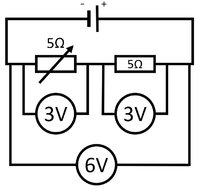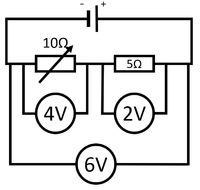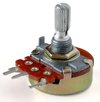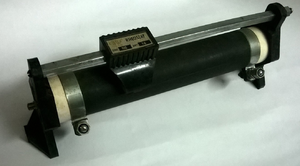# Variable Resistor

## Key Stage 4

### Meaning

A variable resistor is a resistor which can have its resistance changed.In this circuit the variable resistor has a resistance of 0Ω. The potential difference across the fixed resistor is 6V. The current through this circuit is given by$I = \frac{V}{R}$ $$I = \frac{6}{5}$$ $$I = 1.2A$$ In this circuit the variable resistor has the same resistance as the fixed resistor (5Ω). The potential difference across the fixed resistor is 3V. The current through this circuit is given by$I = \frac{V}{R}$ $$I = \frac{6}{10}$$ $$I = 0.6A$$ In this circuit the variable resistor has twice the resistance of the fixed resistor (10Ω). The potential difference across the fixed resistor is 2V. The current through this circuit is given by$I = \frac{V}{R}$ $$I = \frac{6}{15}$$ $$I = 0.4A$$This variable resistor can be turned to change the resistance. This variable resistor is known as a Rheostat. The slider can be moved to change resistance.